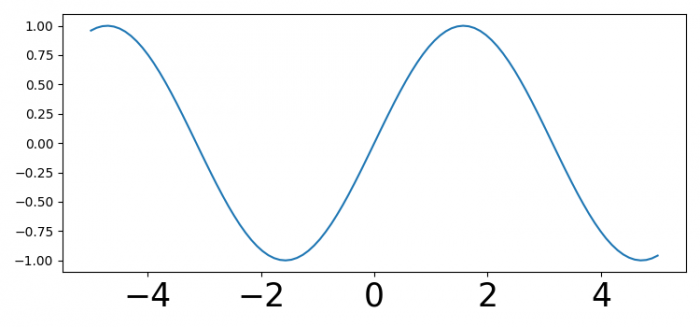# How to change xticks font size in a matplotlib plot?

To change the font size of xticks in a matplotlib plot, we can use the fontsize parameter.

## Steps

• Import matplotlib and numpy.

• Set the figure size and adjust the padding between and around the subplots.

• Create x and y data points using numpy.

• Plot the x and y data points using plot() method.

• Set the font size of xticks using xticks() method.

• To display the figure, use show() method.

## Example

from matplotlib import pyplot as plt
import numpy as np

# Set the figure size
plt.rcParams["figure.figsize"] = [7.50, 3.50]
plt.rcParams["figure.autolayout"] = True

# x and y data points
x = np.linspace(-5, 5, 100)
y = np.sin(x)

plt.plot(x, y)

# Set the font size of xticks
plt.xticks(fontsize=25)

# Display the plot
plt.show()

## Output

It will produce the following output −